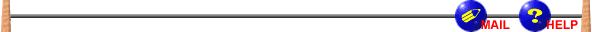Czech ACM Student Chapter
CTU FEE Prague, MFF UK Prague, FEI TU Ostrava
FI MU Brno, FIIT STU Bratislava

## CTU Open 2006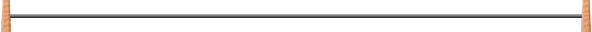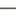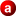## Tree Marking Animals

``` no files available yet ```

A tree marker is an exotic animal that likes numbers and lives on rooted trees. A rooted tree has several nodes, one of them is marked as root. From each node lead several branches to other nodes; one of the branches is a downward branch. There is no downward branch from the root, and each branch of the tree is downward from some node. By following the downward branches from any node, we eventually always reach the root of the tree.

Let us return to tree markers. Tree markers climb through the tree randomly, performing the following operations: Mark the node - they leave a mark in the node in that they currently are. Unmark the node - they remove all the marks from their current node (or do nothing if no mark is there). And finally, jump - the tree marker jumps and falls downward through the tree, until it hits a marked node (possibly the same node it jumped from) or the root of the tree. Your task is to write program that simulates these fascinating creatures.

### Input Specification

The input consists of several instances separated by single empty lines; input of each instance consists of several lines. Each input instance is finished by a line containing a string "All tree markers went for lunch, they will be back at 15:00.". The first line of each instance contains one integer n (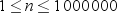), number of nodes of the tree. The nodes of the tree are numbered from 1 to n, where 1 is the root of the tree. The second line of the instance consists of n - 1 numbers a2, ..., an (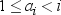) - ai is the number of the node to that the downward branch from the i-th node leads. The remaining lines (except for the last one) contain description of one of the events:

• A string "Mark" followed by an integer k (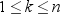) - a tree marker marks the node k.
• A string "Unmark" followed by an integer k () - a tree marker unmarks the node k.
• A string "Jump" followed by an integer k () - a tree marker jumps from the node k.

### Output Specification

For each input instance, output a single line. This line contains several integers separated by single spaces - for each jump event in the instance, write the number of the node in that the tree marked ends his jump.

### Sample Input

```5
1 2 3 4
Mark 2
Mark 3
Jump 5
Mark 4
Jump 5
Unmark 2
Jump 5
Unmark 4
Unmark 3
Jump 5
Mark 4
Jump 4
Unmark 4
Jump 4
All tree markers went for lunch, they will be back at 15:00.

1

Jump 1
Mark 1
Jump 1
All tree markers went for lunch, they will be back at 15:00.
```

### Output for Sample Input

```3 4 4 1 4 1
1 1```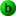## Black Boxes

``` no files available yet ```

A pattern of length k is a sequence a1, a2, ..., ak of k pairwise distinct numbers. Two patterns a1, a2, ..., ak and b1, b2, ..., bk are equivalent if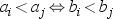for all i and j such that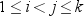. A dictionary is a list of several pairwise nonequivalent patterns.

A black box for a fixed dictionary is a machine that recognizes patterns - given an unknown pattern X, it asks several questions of form "Is the i-th element of the pattern smaller than the j-th one?", and then decides to which of the patterns in the dictionary is X equivalent (in case X is not equivalent to any of the patterns, the black box may answer arbitrarily). The quality of the black box is the maximum number of questions the black box asks for any input.

International Blackbox Machine Corporation manufactures black boxes for various dictionaries, and claims that they ask the least number of questions possible. Your task is to help to verify this claim.

### Input Specification

The input consists of several instances, the instances are separated by single empty lines. The first line of each instance contains integers D and k (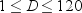,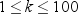) separated by a space, where D is the number of patterns in the dictionary and k is the length of each pattern. Each of the D following lines describes one of the patterns in the dictionary. Each of the lines consist of k distinct integers a1, ..., ak (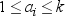) separated by single spaces.

### Output Specification

For each input instance, output a single line containing a single integer Q - the quality of the best possible black box that recognizes the given dictionary.

```4 4
1 2 3 4
1 3 2 4
2 1 4 3
3 1 2 4

1 1
1```

### Output for Sample Input

```2
0```

Comment: It is easy to see that no black box may recognize these patterns by just one question. The black box that recognizes them by two questions may work for example this way: It first asks whether the first element a1 is smaller than the second one a2. If this is the case, it asks whether the second element is smaller than the third one. On the other hand, if a1 > a2, it asks whether the third element is smaller than the fourth one.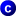## Chocolate Eating

``` no files available yet ```

Alice and Bob play the following game: they start with several chocolate bars, consisting of n1 , n2 , ... , nk pieces. The players alternate on turns, and Alice starts. In each turn, the player may either split one of the bars into two (i.e., replace a bar consisting of n > 1 pieces with two bars consisting of a and b pieces, for any positive integers a and b such that n = a + b), or eat a bar of chocolate that consist of only one piece (if such a piece is available). The game ends when there is no chocolate left. Obviously, the goal of the game is to eat as much chocolate as possible.

### Input Specification

The input consists of several instances; input of each instance consists of single line (whose length is at most 1000000 characters). This line contains a nonempty list of integers n1 , n2 , ... , nk (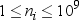), separated by single spaces. The numbers represent the sizes of the chocolate bars.

### Output Specification

For each input instance, output a single line containing a single integer n - the number of pieces of chocolate Alice can eat assuming that both Alice and Bob play the game using the optimal strategy.

`3 2 1`

### Output for Sample Input

`3`

Comment: For example, the game may proceed as follows: Alice eats one piece of chocolate. Bob splits the bar that consists of two pieces. Alice eats one of the created pieces, and Bob the other one. Alice splits the last remaining piece of chocolate to bars with 1 and 2 pieces. Bob eats one piece. Alice splits the last chocolate bar, and each of the players eats one of the created pieces.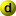## srebmuN desreveR gniddA

``` no files available yet ```

Reversed number is a number written in arabic numerals but the order of digits is reversed. The first digit becomes last and vice versa. For example, if the original number is 1245, the reversed one becomes 5421. Note that all the leading zeros are omitted. That means if the number ends with a zero, the zero is lost by reversing (e.g. 1200, gives 21). Also note that the reversed number never has any trailing zeros.

We need to calculate with reversed numbers. Your task is to add two reversed numbers and output their reversed sum. Of course, the result is not unique, because any particular number is a reversed form of several numbers (e.g., 21 could be 12, 120 or 1200 before reversing). Thus, we must assume that no zeros were lost by reversing (e.g., assume that the original number was 12).

### Input Specification

The input consists of N cases. The first line of the input contains only positive integer N. Then follow the cases. Each case consists of exactly one line with two positive integers separated by space. These are the reversed numbers you are to add.

### Output Specification

For each case, print exactly one line containing only one integer - the reversed sum of two reversed numbers. Omit any leading zeros in the output.

```3
24 1
4358 754
305 794```

### Output for Sample Input

```34
1998
1```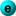## Extra Safety

``` no files available yet ```

We want to build a bridge. A bridge is built by adding joints to it, one by one. We start with a single joint. Then, we always add one joint at a time and connect it with iron bars to some of already-existing joints. These joints are called the base of the new added one. So far, this looks easy. However, we want to build safe bridges. A bridge is considered safe, if all its joints have a solid base, i.e., all joints in the base are directly joined with each other.

Given plans for a new bridge, you are to quickly verify that the bridge is safe.

### Input Specification

The input consists of several instances, separated by single empty lines. The first line of each instance consists of a single number n (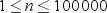) - number of joints of the bridge. The joints are numbered from 1 to n, in the order they are added to the bridge (the initial joint has number 1). Each of the following n - 1 lines describes a joint added to the bridge - the i-th line describes the joint i + 1. Each of these lines starts with a number t (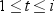), followed by t numbers that identify the joints in the base of the joint i + 1. The total number of the bars in the bridge does not exceed 1000000.

### Output Specification

For each input instance, output a single line containing one integer: the number of the first joint whose base is not solid. In other words, you are to find the first joint that, in the moment when it is being added, is connected to at least two previous joints that are not connected together.

If there is no such joint, output the sentence "The bridge is safe." instead.

```3
1 1
2 1 2

5
1 1
1 1
2 2 3
1 1```

### Output for Sample Input

```The bridge is safe.
4```

Comment: The joint number 4 is connected to joints 2 and 3, which are not connected together directly.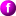## Numbers Factorization

``` no files available yet ```

Factoring numbers into a product of primes is one of the most famous problems in mathematics. Your task is to solve this problem once for all. Remember that a prime is a number that has exactly two natural divisors.

### Input Specification

The input consists of several lines, each of them containing one positive integer number, no bigger than 1000000. The last line contains the number zero, which signals the end of the input.

### Output Specification

For each of the positive numbers, print the number, one space, an equal sign ("`=`"), one more space, and the factorization into prime numbers (factors) on either one or two lines. The factors should be listed in ascending order, separated with one space, small letter "`x`", and one more space. If some factor should appear more than once, use exponents instead, printed on an additional line above the main one. See the Sample Output for an example.

The position of exponents must be exact, each of them beginning one character after the corresponding prime number. The main line (the one with primes) must be filled with extra spaces wherever an exponent appears. Do not display exponents that are equal to 1. If there are no exponents at all, you must not print the additional line.

If the input number is a prime, print it, one space, and the phrase "`is a prime number`". If the input number is 1, output the phrase "`1 is just a one`".

Print one empty line after each factorization or phrase. There may be additional spaces at the end of any line.

```144
13
10
1
65535
999808
0```

### Output for Sample Input

```       4    2
144 = 2  x 3

13 is a prime number

10 = 2 x 5

1 is just a one

65535 = 3 x 5 x 17 x 257

7
999808 = 2  x 73 x 107
```

Comment: As you can see, exact formatting is important in this problem. Read the instructions carefully and follow them exactly. Otherwise, you will get a presentation error.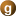## Random Generators

``` no files available yet ```

A pseudorandom generator is a function that produces sequence of seemingly random integers laying in some range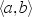(where a and b are nonnegative integers). Given two pseudorandom generators, we want to combine them together. The easiest way is to take the values of the combined generator as xor of the values of the two input generators. The problem is to decide what is the range of possible values of the new generator, i.e., given two ranges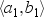and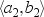, find the rangesuch that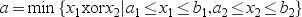and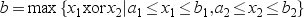.

### Input Specification

The input consists of several instances; input of each instance consists of a single line. This line contains four numbers a1, b1, a2 and b2 (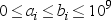), separated by single spaces. The value ranges of the two input generators areand.

### Output Specification

For each input instance, output a single line containing two integers a and b separated by a single space, giving the value rangeof the combined generator.

### Sample Input

```5 5 10 10
5 6 6 7```

### Output for Sample Input

```15 15
0 3 ```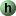## Ball Lightning -- Help with the Moving

``` no files available yet ```

This time, we need you to help us with moving our offices. Currently, we live in n offices, numbered from 1 to n, and every office is occupied. Our management however decided to change the arrangement, and presented us with plan of who of us should move where. After some negotiation, we have achieved the state in that everyone is supposed to move to exactly one office, and no two people must move to the same office. However, to make the move more efficient, we need to do it in two phases. In the first phase, each day just two persons swap their offices. The second phase is done in just one day, in that everyone moves to some office different from his/hers current one, and additionally all the moves form just one cycle (i.e., they can be described as an ordering k1, ..., kn of numbers from 1 to n such that the person in the ki-th office moves to the ki+1-th office (the person in the kn-th office moves to the k1-th office). Your task is to prepare the first phase in such a way that the move can be achieved in the shortest possible time.

### Input Specification

The input consists of several instances; input of each instance consists of single line. This line contains an integer n (), followed by n pairwise distinct integers m1, ..., mn (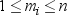). These number mean that the person in the i-th office should move to the mi-th office.

### Output Specification

For each input instance, output a single line containing one integer - the number of days necessary to move all the persons, including the one day for the second phase.

### Sample Input

`5 1 2 3 5 4`

### Output for Sample Input

`4`

Comment: The first day, persons in offices 1 and 2 exchange them. The second day, the offices 2 and 3 exchange. On the third day, persons in offices 3 and 4 exchange their places. Finally, on the fourth day, everyone moves to their scheduled offices.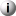## Intercal

``` no files available yet ```

Your task is to write one essential part of the Intercal compiler (Intercal is a well-known programming language). Your program should write in an integer from input, calculate its unary and, and read out the result.

An unary and of a 32-bit nonnegative integer that can be written in binary as b0b1 ... b31 is the 16-bit integer that can be written in binary as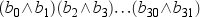. For example, unary and of 13 = 0000 ... 1101 is 0000 ... 10 = 2.

### Input Specification

The input consists of several instances; input of each instance consists of a single line. Each line contains one integer number x (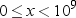), written in English. I.e. 0 is "zero", 1 is "one", 2 is "two", 3 is "three", 4 is "four" 5 is "five", 6 is "six", 7 is "seven", 8 is "eight", 9 is "nine", 10 is "ten", 11 is "eleven", 12 is "twelve", 13 is "thirteen", 14 is "fourteen", 15 is "fifteen", 16 is "sixteen", 17 is "seventeen", 18 is "eighteen", 19 is "nineteen", 20 is "twenty", 30 is "thirty", 40 is "forty", 50 is "fifty", 60 is "sixty", 70 is "seventy", 80 is "eighty", 90 is "ninety".

A number between 21 and 99 whose number of units is not equal to zero is expressed as composition of terms for the tens and the units, e.g., 56 is "fifty six". A number between 100 and 999 is expressed by the term for hundreds, followed by "hundred", followed by the term for the remainder of the number modulo 100 if it is not zero, preceded by "and", e.g., 124 is "one hundred and twenty four" and 500 is "five hundred".

A similar construction is used for multiples of 1000 (using word "thousand") and 1000000 (using word "million"). In these cases, the possible remainder of the number is not preceded by "and", e.g., 999999999 is "nine hundred and ninety nine million nine hundred and ninety nine thousand nine hundred and ninety nine" and 500000905 is "five hundred million nine hundred and five".

### Output Specification

For each input instance, output a single line containing the unary and of the input number. The number should be output in Roman numerals. It is guaranteed that the output will never be zero, nor greater than 3999. Roman numerals are represented by a string consisting of characters "I", "V", "X", "L", "C", "D" and "M". These symbols represent the decimal values 1, 5, 10, 50, 100, 500 and 1000 respectively. To represent other values, these symbols, and multiples where necessary, are concatenated, with the smaller-valued symbols written further to the right. For example, the number 3 is represented as "III", and the value 73 is represented as "LXXIII".

The exceptions to this rule occur for numbers having units values of 4 or 9, tens values of 40 or 90, and hundreds values of 400 and 900. For these cases, the Roman numeral representations are "IV" (4), "IX" (9), "XL" (40), "XC" (90), "CD" (400) and "CM" (900). So the Roman numeral representations for 24, 39, 44, 49, and 94 are "XXIV", "XXXIX", "XLIV", "XLIX", and "XCIV", respectively. At most three consecutive characters "I", "X", "C" and "M" may appear in the numeral, and each numeral contains characters "V", "L", "D" at most once. It is forbidden to use e.g. "IC" for 99.

### Sample Input

```thirteen
ninety nine million ninety nine thousand nine hundred and ninety nine```

```II
MMLI```